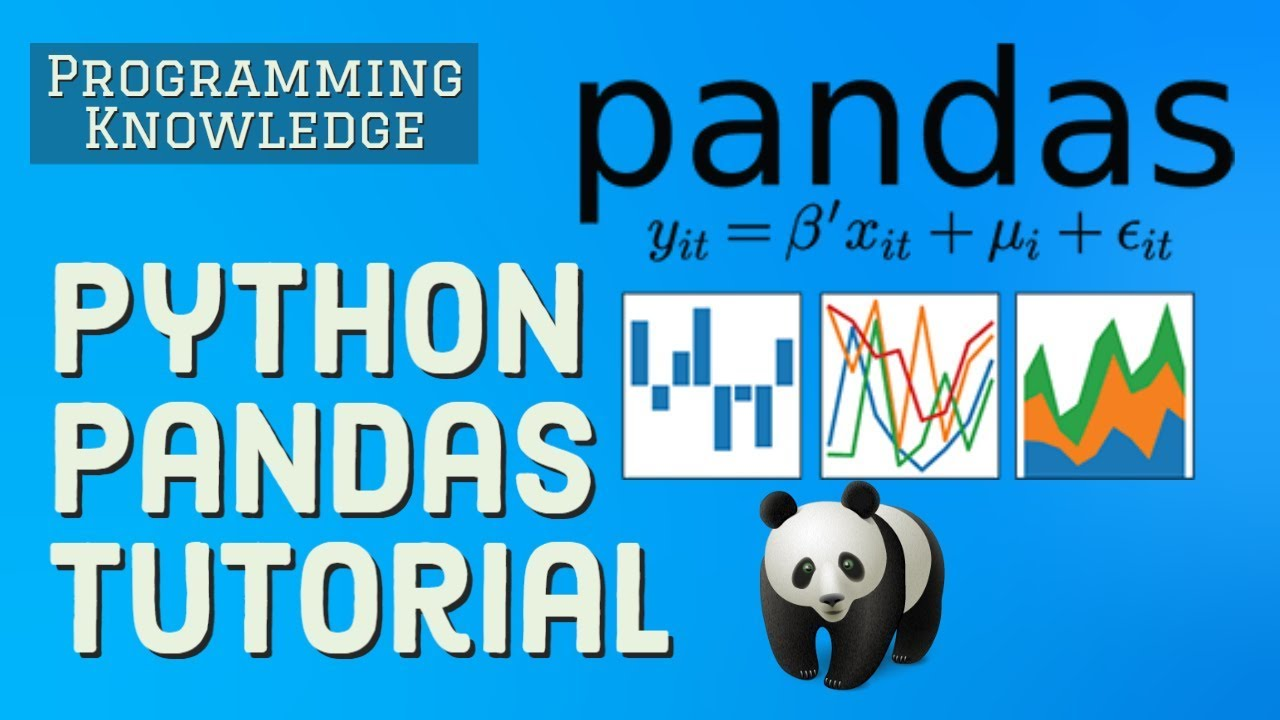# Python Pandas Tutorial | Data Science For Beginners With Python PandasWelcome to this course on Data Science For Beginners With Python Pandas. Learn how Perform a Many of data operations in Python's popular Pandas library.

Welcome to this course on Data Science For Beginners With Python Pandas. Learn how Perform a Many of data operations in Python's popular Pandas library.

🐼 Content 🐼 00:00:01 1 - Introduction to Data Science + Python Environment Setup 00:08:57 2 - Importing Datasets in Pandas and Removing Junk 00:24:23 3 - Copying, Selecting, Indexing data from Pandas Dataframes 00:40:22 4 - Pandas dataframe Counting, Summarized view, Data Types 00:54:26 5 - Cleaning and Converting the Pandas Dataframe columns 01:07:13 6 - If Else and Looping Constructs in Pandas Part 1 01:19:16 7 - If Else and Looping Constructs in Pandas Part 2 01:24:29 8 - Exporting data to file, Aggregate Statistics, Describing 01:35:53 9 - Calculate Frequency Tables, Two way Tables... 01:45:53 10 - Conditional Probability and Correlation in Pandas 01:57:20 11 - Dataframe Visualization using Matplotlib and Seaborn 02:11:23 12 - Numerical and Categorical variables with Matplotlib 02:23:53 13 - Filling Missing Categorical values in Pandas Dataframes Accuracy measures in Data Mining

## Basic Data Types in Python | Python Web Development For Beginners

In the programming world, Data types play an important role. Each Variable is stored in different data types and responsible for various functions. Python had two different objects, and They are mutable and immutable objects.

## Python for Data Science - Course for Beginners (Learn Python, Pandas)

This Python data science course will take you from knowing nothing about Python to coding and analyzing data with Python using tools like Pandas, NumPy, and ...

## Python for Data Science - Learn Python, Pandas, NumPy, Matplotlib

Python tutorial for Data Science - Learn Python, Pandas, NumPy, Matplotlib, will take you from knowing nothing about Python to coding and analyzing data with Python using tools like Pandas, NumPy, and matplotlib. This is a hands-on course and you will practice everything you learn step-by-step.

## Python Pandas Tutorial - Data Analysis with Python Pandas

Python Pandas Tutorial will help you get started with Python Pandas Library for various applications including Data analysis. Introduction to Pandas. DataFrames and Series. How To View Data? Selecting Data. Handling Missing Data. Pandas Operations. Merge, Group, Reshape Data. Time Series And Categoricals. Plotting Using Pandas

## Data Science Course in Dallas

Become a data analysis expert using the R programming language in this [data science](https://360digitmg.com/usa/data-science-using-python-and-r-programming-in-dallas "data science") certification training in Dallas, TX. You will master data...